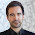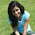## Sunday, July 7, 2013

### change in weight of cars plot

Based on last week's faster algorithm I wanted to finish with car weights. Unfortunately a fail again. By now it is a fail of myself, it needs a bit more dedication and grunt than I am willing and able to give for this blog. This week I added some extra functions around the existing functions so I could harvest final results pretty easily. But the results seemed a bit odd at places, I ran the same again, the second time around they were a bit different. Nothing that seems unsolvable with a bit more attention, manually checking if stable sampler has been obtained, maybe tune the number of normal distributions which make the combined distribution.
Having said the negative, the pictures can be interpreted. The car market has changed from a double peaked distribution to a three peak distribution. Sales in recent years are mostly in the lowest weight category. The weight of cars in this category is slowly increasing though.

#### Data

Data were obtained as described here.  I made an additional plot of the weights. These are the raw data weighted by the width of the categories.
lweight4 <- weight2[weight2\$RefYear == weight2\$BuildYear+1,]
weight4\$lower <- lweightcats[weight4\$Onderwerpen_2]
weight4\$upper <- uweightcats[weight4\$Onderwerpen_2]
datashow <- expand.grid(year=2000:2012,
weight=seq(500,2000,by=20))
datashow\$y <-0
for (ii in 1:nrow(weight4)) {
datashow\$y[datashow\$weight>=weight4\$lower[ii]
& datashow\$weight<=weight4\$upper[ii]
& datashow\$year==weight4\$BuildYear[ii]] <-
weight4\$Waarde[ii]*100/(weight4\$upper[ii]-weight4\$lower[ii])
}
library(lattice)
levelplot(y ~ weight+ year , data=datashow,   col.regions=
colorRampPalette(c('white','yellow','green','blue','purple','red'))
)
xyplot(y ~ weight | factor(year) , data=datashow,type='l')

#### Modelling.

I worked on the previous code to obtain something which was very short and wrapped in a lapply. This means I had to trust the outcome, which I thought was reasonable.
la <- lapply(2000:2012,function(x) {
weight <- makdata(x)
weightlims <- c(0,weight\$upper)
update <- updater(weight)
dist <- update2line(weight,update)
dist\$year <- x
dist
}
)
rbla <- do.call(rbind,la)
levelplot(y ~ x+ year  , data=rbla[rbla\$x %in% seq(700,1600,10),],
col.regions=
colorRampPalette(c('white','yellow','green','blue','purple','red'))
The nice thing about this plot is that it clearly shows three peaks within each year and a slow increase of the locations of the years. 2009 looks a bit odd though. The lowest category existed maybe at 2003, but really took off in 2010. In 2009 it also seems the heaviest cars got less popular, but this seems to be reverting slightly in 2011.

#### model flaws

So I did the calculations again, resulting in rbla2, because I found 2009 odd compared to the rest. For comparison the next plot:
rbla\$sim <- 1
rbla2\$sim <- 2
rblaall <- rbind(rbla,rbla2)
xyplot(y ~ x |  factor(year) ,group=sim, type='l', data=rblaall[rblaall\$x %in% seq(700,1600,10),])
A number of panes have separate lines, indicating different results. It seems the results of 2000, 2002 and 2009 are different, hence needing a bit more attention

#### R-code

weight2 <- weight1[!is.na(weight1\$Waarde),]
weight2\$BuildYear <- as.numeric(sub('Bouwjaar ','',as.character(weight2\$Bouwjaren)))
weight2\$RefYear <- as.numeric(sub(', 1 januari','',as.character(weight2\$Peildatum)))
weightcats <- levels(weight2\$Onderwerpen_2)
weightcats <- gsub('en meer','and more',weightcats)
levels(weight2\$Onderwerpen_2) <- weightcats
lweightcats <- as.numeric(gsub('( |-).*\$','',weightcats))
uweightcats <- as.numeric(gsub('(^[0-9]+-)|([ [:alpha:]]+\$)','',weightcats))
uweightcats[uweightcats==2451] <- 3500

nspacejump1 <- function(x,i,sd) {
xold <- log(x[i]/(1-x[i])) + rnorm(1,0,sd)
xnew <- 1/(1+exp(-xold))
x <- x/sum(x[-i])*(1-xnew)
x[i] <- xnew
x
}

curldev5 <- function(counts,curmodel,pemodel) {
dsd <- sum(dnorm(curmodel\$sd,300,300,log=TRUE))
prop.exp <- pemodel  %*% curmodel\$w
prop.exp <-  prop.exp/sum(prop.exp)
dsd + lgamma(sum(counts\$Waarde) + 1) + sum(counts\$Waarde * log(prop.exp) - counts\$lgam)
#dmultinom(counts\$Waarde,prob=prop.exp,log=TRUE)
}

version6 <- function(weight3,curmodel,niter) {
pemodel <- matrix(0,nrow=22,ncol=nrow(curmodel))
pemodel2 <- pemodel
for (i in 1:nrow(curmodel)) {
pn <- pnorm(weightlims,curmodel\$mean[i],curmodel\$sd[i])
pemodel[,i] <- (pn[-1]-pn[-23])
}
cnow <- curldev5(weight3,curmodel,pemodel)
ndist <- nrow(curmodel)
result <- matrix(nrow=ndist*niter,ncol=3*ndist+1)
index <- 1
for (iter in 1:niter) {
for (dist in 1:ndist) {
nowmodel <- curmodel
nowpe <- pemodel
result[index,] <- c(curmodel\$mean,curmodel\$sd,curmodel\$w,cnow)
curmodel\$mean[dist] <- curmodel\$mean[dist] + rnorm(1,0,1)
curmodel\$sd[dist] <- curmodel\$sd[dist] * runif(1,1/1.01,1.01)
curmodel\$w <- nspacejump1(curmodel\$w,dist,.1)
pn <- pnorm(weightlims,curmodel\$mean[dist],curmodel\$sd[dist])
pemodel[,dist] <- (pn[-1]-pn[-23])
cnew <- curldev5(weight3,curmodel,pemodel)
# cat(c(cnow,cnew,'\n'))
if (exp(cnew-cnow) > runif(1) ) cnow <- cnew
else {curmodel <- nowmodel
pemodel <- nowpe }
index <- index + 1
}
}
return(list(result=result,curmodel=curmodel))
}
curmodel5 <- data.frame(
mean=c( 892.0141263, 1.417520e+03, 1306.8248062, 1.939645e+03,1000),
sd  =c(  99.5660288, 2.129247e+02,  194.2452168, 1.684932e+02,100),
w   =c(   0.2252041, 4.384217e-02,    0.6125014, 1.845233e-02,.1))

makdata <- function(xxxx) {
weightxxxx <- weight2[weight2\$RefYear==xxxx+1 & weight2\$BuildYear==xxxx,c(2,6)]
weightxxxx\$lower <- lweightcats[weightxxxx\$Onderwerpen_2]
weightxxxx\$upper <- uweightcats[weightxxxx\$Onderwerpen_2]
weightxxxx\$lgam <- lgamma(weightxxxx\$Waarde + 1)
weightxxxx
}

updater <- function(weight,nburnin=200000,nsample=10000) {
update  <- version6(weight,curmodel5,nburnin)
update <- version6(weight,update\$curmodel,nsample)
plot(update\$result[,ncol(update\$result)],type='l')
return(update)
}

update2line <- function(weight,update) {
integral <- sum((weight\$upper-weight\$lower)*weight\$Waarde)
x <- seq(0,3500,10)
ysum <- rep(0,length(x))
for(i in seq(1,10000,100)) {
ndist <- nrow(update\$curmodel)
y <- rep(0,length(x))
newmodel <- data.frame(mean = update\$result[i,1:ndist],
sd=update\$result[i,ndist+(1:ndist)],
w=update\$result[i,2*ndist+(1:ndist)])
for (ir in 1:nrow(newmodel))
y <- y +  newmodel\$w[ir]*dnorm(x,newmodel\$mean[ir],newmodel\$sd[ir])
ysum <- ysum+y
}
data.frame(x=x,y=integral*ysum/sum(y))
}

1.My friend mentioned to me your blog, so I thought I’d read it for myself. Very interesting insights, will be back for more! check our our website here

2.You have raised an important issue..Thanks for sharing..I would like to read more current affairs from this blog..keep posting.. sell my car

3.My friend mentioned to me your blog, so I thought I’d read it for myself. Very interesting insights, will be back for more! used Holden Commodores

4.This comment has been removed by a blog administrator.

5.This comment has been removed by a blog administrator.